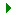Search    VB VB.net C# ASP.net ASP SQL Server VC++ My Links Networking ORACLE MySQL PHP Unix/Linux WinNT/2k/2003 Flash Maya 3D max Photoshop DB2 C/C++/ASM Microcontroller Circuit Design JAVA Wireless/Mobile JScript     Articles from this site only Links only Articles with downloads Articles with screenshot(s)  Code/Page 10 20 30User Login Email Password Remember MeForgot the Password?Services
» Web Development
» Maintenance
» Data Integration/BI
» Information ManagementProgramming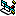VB (1648)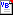VB.net (736)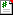C# (15)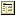ASP.net (779)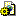ASP (41)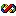VC++ (25)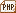PHP (0)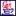JAVA (4)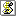JScript (5)Database
 » SQL Server (708) » ORACLE (5) » MySQL (0) » DB2 (0)Automation
 » C/C++/ASM (11) » Microcontroller (1) » Circuit Design (0)OS/Networking
 » Networking (5) » Unix/Linux (1) » WinNT/2k/2003 (8)Graphics
 » Flash (0) » Maya (0) » 3D max (0) » Photoshop (0)Links
 » ASP.net (2) » PC Interfacing (1) » Networking (4) » SQL Server (4) » VB (23) » VB.net (4) » VC (3) » HTML/CSS/JavaScript (10)Tools
» Regular Expr Tester
» Free Tools

 (Page 1 of 2)55 Result(s) found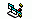A simple expression evaluator Total Hit (2415) While any Windows user could pop up the Calculator accessory to perform any type of math calculations, it would be great if you could offer him or her the capability to do simple math from within your application. This is a very simple expression evaluator function that does it: This evaluator i ....Read More RatingThe "And" operator is faster than "Mod" Total Hit (3480) Using the "And" operator instead of "Mod" may speed up your program under certain situations. Use the And operator instead of Mod when the divisor is a number in the form 2^N. For instance, there are two methods to extract the least significant byte in an Integer: «Code LangId=1» lowByte% = valu ....Read More Rating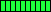Avoid Integer Overflow Total Hit (2148) When working with integer expressions there is often the risk of raising the "Overflow" error. More specifically, there can be two occasions when this frequently occurs: When you multiply or add Integer values and the result exceeds 32,767. When you create generic string routines that iterate ....Read More RatingConvert Hexadecimal numbers Total Hit (3389) While Visual Basic offers the Hex\$ function that converts a decimal value into its hexadecimal equivalent number, it seems that the inverse function is missing. Not true. Try out this one-liner: «Code LangId=1» Function HexToDec(HexValue As String) As Long HexToDec = Val("&H" & HexValue) ....Read More RatingCounting Bits Total Hit (2504) It seems that the only way to count the number of 1's or 0's in a binary value is creating a loop that iterates on all the 16 or 32 bits of the number. There is, however, a faster algorithm: «Code LangId=1» Function BitCount (ByVal number As Long) As Integer Dim bits As Integer, temp As Long ....Read More RatingDetermine whether the app is running on a flawed Pentium CPU Total Hit (2092) Here's a simple test that you can use to determine whether the application is running on a system equipped with a Pentium CPU affected by the FDIV bug: «Code LangId=1» ' return True if the CPU suffers from the FDIV bug Function IsBuggedPentium() As Boolean IsBuggedPentium = ((1 / 3221224 ....Read More RatingEvaluate the integer equal or higher than a given value Total Hit (2212) The VBA language offers the Int() function, which returns the integer equal or lower than a given value, but lacks a similar function that returns the integer equal or higher than a given value. You can remedy with the following function: «Code LangId=1» ' Returns the integer equal or higher tha ....Read More RatingManually coerce to Long all Integer expressions that might overflow Total Hit (2283) This is something that expert VB developers know very well, yet every know and then an otherwise perfect VB app stops with a fatal overflow error because of Integer overflow. Consider the following code: «Code LangId=1» Dim LongVariable As Long LongVariable = 256 * 256 «/Code» This raises th ....Read More RatingUnderstanding the "Allow Unrounded Floating Point Operations" option Total Hit (2324) The Microsoft manuals preach that all the compiler options in the Advanced Optimization dialog box are to be considered unsafe, in that they might lead to incorrect results (or just program crashes!). This is true for most of them, but often one of such options - namely, the "Allow Unrounded Floatin ....Read More RatingUndocumented behavior of the CInt() function Total Hit (2467) The CInt() function rounds to the nearest integer value. In other words, CInt(2.4) returns 2, and CInt(2.6) returns 3. This function exhibits an under-documented behavior when the fractional part is equal to 0.5. In this case, this function rounds down if the integer portion of the argument is e ....Read More Rating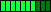Use integer division operator Total Hit (2183) Use "\" instead of "/" when performing divisions between Integers. The "/" operator returns a Single value, therefore the seemingly efficient line «Code LangId=1» C% = A% / B% «/Code» actually requires three implicit conversions, two for converting the operands from Integer to Single (to pre ....Read More RatingAny2Dec - Convert from any numeric base to decimal Total Hit (2586) «Code LangId=1»' convert from any base to decimal ' BASE can be in the range 2-36 Function Any2Dec(ByVal otherBaseNumber As String, ByVal base As Integer) As Long Dim index As Long Dim digits As String Dim digitValue As Long ' check base If base < 2 Or base > 36 T ....Read More RatingASin, ACos, ACot, ASec, ACsc - Missing inverse trig functions Total Hit (2461) «Code LangId=1»' arc sine ' error if value is outside the range [-1,1] Function ASin(value As Double) As Double If Abs(value) <> 1 Then ASin = Atn(value / Sqr(1 - value * value)) Else ASin = 1.5707963267949 * Sgn(value) End If End Function ' arc cosine ' er ....Read More RatingASinH, ACosH, ATanH, ACotH, ASecH, ACscH - Hyperbolic inverse trig functions Total Hit (2963) «Code LangId=1» ' hyperbolic arc sine Function ASinH(value As Double) As Double ASinH = Log(value + Sqr(value * value + 1)) End Function ' hyperbolic arc cosine ' error if NUMBER is inside the range [-1,1] Function ACosH(value As Double) As Double ACosH = Log(value + Sqr(value ....Read More RatingAtn2 - Arc tangent of Y/X Total Hit (2647) «Code LangId=1»' arc tangent of Y/X - returns values in all four quadrants Function Atn2(x As Double, y As Double) As Double If x = 0 Then Atn2 = Sgn(y) * 1.5707963267949 ElseIf x > 0 Then Atn2 = Atn(y / x) Else Atn2 = Atn(y / x) + 3.14159265358979 * Sgn ....Read More Rating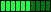Base Conversion module - A module to convert numbers between any bases Total Hit (3396) «Code LangId=1»'----------------------------------------------------------------- ' Module: mBases ' (C) 2000 Trinet Ltd, http://www.trinet.co.uk ' Author: R. Deeming (richard@trinet.co.uk) ' ' Purpose: To provide simple conversion between different ' number ba ....Read More RatingBin - Convert from decimal to binary Total Hit (2182) «Code LangId=1»' convert from decimal to binary ' if you pass the Digits argument, the result is truncated ' to that number of digits ' Function Bin(ByVal value As Long, Optional digits As Long = -1) As String Dim result As String, exponent As Integer ' this is faster than creating t ....Read More RatingBinToDec - Convert from binary to decimal Total Hit (3318) «Code LangId=1»' convert from binary to decimal ' Function BinToDec(value As String) As Long Dim result As Long, i As Integer, exponent As Integer For i = Len(value) To 1 Step -1 Select Case Asc(Mid\$(value, i, 1)) Case 48 ' "0", do nothing Case 4 ....Read More RatingBitClear - Clear a bit in a value Total Hit (2078) «Code LangId=1»Function BitClear(ByVal value As Long, ByVal bit As Long) As Long ' simply AND with the negation of the bit mask ' Range checking is performed in Power2() BitClear = (value And Not Power2(bit)) End Function ' Raise 2 to a power ' the exponent must be in the range [ ....Read More RatingBitCount - The number of "1" bits in a number Total Hit (2014) «Code LangId=1» ' The number of 1's in a binary number ' ' This routine is based on the following property ' of binary numbers: n And (n-1) always clears the ' least significant "1" bit in the number Function BitCount (ByVal number As Long) As Integer Do While number number = ....Read More RatingBitSet - Set a bit in a number Total Hit (2220) «Code LangId=1»' Set a bit in a value ' ' NOTE: requires Power2() Function BitSet(ByVal value As Long, ByVal bit As Long) As Long ' simply OR with the bit mask ' Range checking is performed in Power2() BitSet = (value Or Power2(bit)) End Function ' Raise 2 to a power ' the e ....Read More RatingBitTest - Test the value of a bit Total Hit (2814) «Code LangId=1»' Test the value of a bit ' ' NOTE: requires Power2() Function BitTest(ByVal value As Long, ByVal bit As Long) As Boolean ' simply AND with the bit mask ' Range checking is performed in Power2() BitTest = (value And Power2(bit)) End Function ' Raise 2 to a po ....Read More RatingBitToggle - Invert a bit in a value Total Hit (2281) «Code LangId=1»' Toggle a bit in a value ' ' NOTE: requires Power2() Function BitToggle(ByVal value As Long, ByVal bit As Long) As Long ' simply XOR with the negation of the bit mask ' Range checking is performed in Power2() BitToggle = (value Xor Power2(bit)) End Function ....Read More RatingCComplexNumber - A class for dealing with complex numbers Total Hit (2150) «Code LangId=1»Option Explicit '------------------------------------------ ' A class for dealing with complex numbers '------------------------------------------ ' The main properties Public Real As Double Public Imaginary As Double ' Initialize this complex number ' (returns Me) Fu ....Read More RatingCeiling - The integer equal or higher than a given value Total Hit (2084) «Code LangId=1»' Returns the integer equal or higher than its argument Function Ceiling(Number As Double) As Long Ceiling = -Int(-Number) End Function «/Code» RatingCelsiusToFahrenheit, FahrenheitToCelsius - Convert temperature values Total Hit (2188) «Code LangId=1»' Returns the integer equal or higher than its argument Function Ceiling(Number As Double) As Long Ceiling = -Int(-Number) End Function «/Code» RatingCombinations - The number of combinations of N objects in groups of N Total Hit (1954) «Code LangId=1»' number of Combinations of N objects in groups of M ' ' Note: requires the FACTORIAL routine Function Combinations(ByVal Objects As Long, ByVal GroupSize As Long) As Double Combinations = (Factorial(Objects) / Factorial(Objects - GroupSize)) / _ Factorial(GroupSize ....Read More RatingCot, Sec, Csc - Missing trig functions Total Hit (2477) «Code LangId=1»' Cotangent of an angle Function Cot(radians As Double) As Double Cot = 1 / Tan(radians) End Function ' Secant of an angle Function Sec(radians As Double) As Double Sec = 1 / Cos(radians) End Function ' cosecant of an angle Function Csc(radians As Double) As ....Read More RatingCrc16 - Evaluate the 16-bit CRC of an array of bytes Total Hit (3458) «Code LangId=1»Option Explicit ' Evalutate the 16-bit CRC (Cyclic Redundancy Checksum) of an array of bytes ' ' If you omit the second argument, the entire array is considered Function Crc16(cp() As Byte, Optional ByVal Size As Long = -1) As Long Dim i As Long Dim fcs As Long Static ....Read More RatingDec2Any - Convert a decimal number to any other base Total Hit (2518) «Code LangId=1»' convert a number to any base ' BASE can be in the range 2-36 Function Dec2Any(ByVal number As Long, ByVal base As Integer) As String Dim index As Long Dim digits As String Dim digitValue As Long ' check base If base < 2 Or base > 36 Then Err.Raise ....Read More Rating(Page 1 of 2)55 Result(s) found 1 2